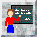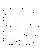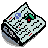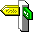Teach-in '99 : Center of Mass & Base of Support

For stability, the body center of mass (CoM) must remain within the base of support (BoS). However, in gait it passes outside the BoS twice every gait cycle - it passes in front of the trailing toe in early swing, and is behind the leading heel in late swing:

In the animation shown, the CoM is the small green dot in the pelvis. The graph below shows the horizontal distance between this and the nearest limit of the BoS. Vicon BodyBuilder modelling software was used to calculate the CoM, using the COMpost model by Michael Eames & Richard Baker (download from the CGA BodyBuilder Model Database). The distance between CoM and BoS was calculated using the following BodyBuilder script:

CoMBoS = 0

{*Left foot down - measure distance between CoM and left toe or heel*}
if (LTOE(3) < 50  or LHEE(3) < 60) then
if (LTOE(3) < 50 and COFM(1) > LTOE(1)) then
CoMBoS = COFM(1) - LTOE(1)
ELSif (LHEE(3) < 60 and COFM(1) < LHEE(1)) then
CoMBoS = COFM(1) - LHEE(1)
Endif

{*Right foot down - measure distance between CoM and right toe or heel*}
Elseif (RTOE(3) < 50  or RHEE(3) < 60) then
if (RTOE(3) < 50 and COFM(1) > RTOE(1)) then
CoMBoS = COFM(1) - RTOE(1)
ELSif (RHEE(3) < 60 and COFM(1) < RHEE(1)) then
CoMBoS =  COFM(1) - RHEE(1)
Endif
Endif

{*Double Support - CoM - BoS is zero at these times*}
if (LTOE(3) < 50  or LHEE(3) < 60) and (RTOE(3) < 50 or RHEE(3) < 60)
CoMBoS = 0
Endif

OUTPUT(CoMBoS)

Note that the foot is deemed to be on the floor if the toe and/or heel markers are lower than 50 or 60 mm, respectively. These values were found emprically, and due to the consequent deadband zones, the CoM-BoS is probably underestimated.

It's of interest that the initial slope of the spikes is triangular, suggesting that the CoM is moving at constant velocity during these times of instability. Moreover, this velocity is precisely the same as that during the rest of the gait cycle.Questions

• Does this data mean that the body is in freefall during these periods?
• If so, how does the body maintain near constant velocity of the CoM?
• What are the clinical implications, e.g. for falls in the elderly?
Email your answers to [n/a]What we said on the CGA listBack to Teach-in page